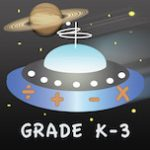@Reks: Educational iOS Applications

## 2nd Grade Printable Math Quiz Workshe...You can download a number of math practice worksheets designed for Grade 2  Elementary School. The practice worksheets are based on Math Essentials textbook and math common core standards. Unit 1 covers the following concepts: add and subtract numbers up to 20 add three or four numbers find partners and totals write equations for math mountains tell […]

## NEW App! Astro Math: Grades K-3Astro Math: Grades K-3 by @Reks is a reliable follow-up resource for common core standardized lessons. The app includes math facts covering kindergarten through 3rd grade school curriculum.

## Astro Math: Grades K-3The app is a reliable follow-up resource for common core standardized lessons. Astro Math: Grades K-3 by @Reks includes math facts covering kindergarten through 3rd grade school curriculum. By protecting each planet individually a student goes through levels practicing grade-level appropriate math skills (counting, addition, subtraction, multiplication, division). The student collects coins upon successfully completed missions and […]